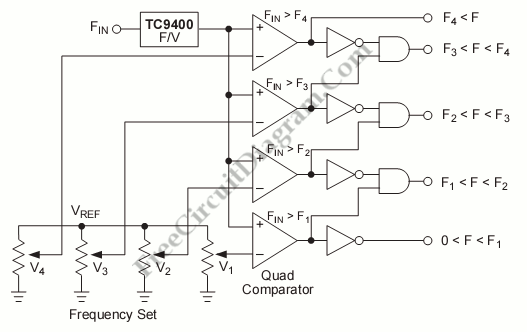# Frequency/Tone Decoder with TC9400 FVCAnother application of FVC (frequency-to-voltage converter) is tone/frequency decoder. This circuit is used to determine the frequency band of an oscillation signal. This circuit is used in many application like determines the frequency band in the signal and remote control where the frequency band corresponds to a different command. This circuit uses TC9400 F/V converter to convert the frequency to voltage because the frequency must be converted to proportional analog voltage before can be detected. Here is the schematic diagram of the circuit:

Beside TC9400 F/V converter, this circuit also uses the quad comparators. It used to detect when the frequency limits is exceeded by the voltage(frequency). The frequency is indicated by the logical “1” at any of the five output. [Circuit’s schematic diagram source: Microchip Application Note]